﻿ WHERE Clause in SQL - WHERE in Clause SQL

# WHERE Clause in SQL

## SQL offers various constructs to help users interact with the stored data effectively. One of these constructs is the SQL WHERE clause in which filtered rows are returned from a SELECT, UPDATE, or DELETE statement.

Here we are going to explain the ins and outs of the SQL WHERE clause and where in SQL we should use the SQL Where Clause.

You can create a free account on FreeASPHosting.net, create a free SQL Server Database and start practicing the SQL Where clause!

## KEY TAKEAWAYS:

1. The SQL WHERE clause is used for filtering rows returned from a SELECT, UPDATE, or DELETE statement based on specified conditions.
2. The SQL WHERE clause is commonly used with the SQL SELECT statement to retrieve specific data that meets certain criteria. It allows you to specify conditions such as less than (<), greater than (>), equal to (=), and more.
3. The WHERE IN clause SQL is a shorthand for multiple OR conditions. It allows you to specify multiple values in the WHERE clause, making it easier to filter data based on different possibilities.
4. The SQL WHERE NOT IN clause is used to exclude certain records based on their column values. It allows you to specify values that should not be included in the result set.
5. The SQL WHERE LIKE clause is used when you want to match a pattern. It is often used with wildcard characters like the percent sign (%) to search for records that match a specific pattern or contain a specific substring.
6. The SQL CASE WHEN statement is used to perform conditional logic and provide different output values based on specified conditions. It allows you to evaluate a series of conditions.

## SQL SELECT Statement with WHERE Clause

The most basic usage of the WHERE SQL clause is with the SQL SELECT statement. It retrieves data from a database. However, in many cases, you would want to retrieve only specific data that meets certain criteria. This is where the SQL WHERE clause comes into play.

Let's consider an example using the following 'Orders' table:Now, suppose we want to find all orders above \$1000. The SQL Select Where clause would look like this:

``````SELECT *
FROM Orders
WHERE Amount > 1000;

``````

And the output of SQL where in the table would be: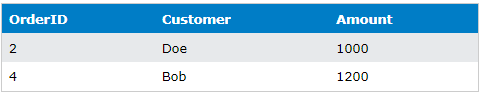## WHERE IN Clause SQL

The WHERE IN clause SQL is a shorthand for multiple OR conditions. It allows us to specify multiple values in the SQL WHERE clause.

Consider the same 'Orders' table. If we want to find the orders made by John and Alice, we could use the IN clause:

``````SELECT *
FROM Orders
WHERE Customer IN ('John', 'Alice');

``````

SQL where output: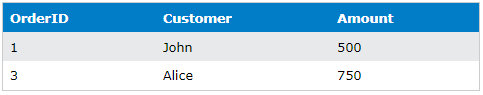## SQL WHERE NOT IN

The SQL WHERE NOT IN clause is used to exclude certain records based on their column values.

To find orders not made by John or Alice, the SQL query would be:

``````SELECT *
FROM Orders
WHERE Customer NOT IN ('John', 'Alice');

``````

Where SQL output:## SQL WHERE LIKE

The SQL WHERE LIKE clause is used in SQL where query when you want to match a pattern. It's often used with a wildcard character, percent sign (%).

Assume that we want to find customers whose names start with 'A'. The SQL where query would be:

``````SELECT *
FROM Orders
WHERE Customer LIKE 'A%';

``````

The output of SQL where clause in the table would be:And if we want to find customers whose names end with “e”. The SQL where query would be:

``````SELECT *
FROM Orders
WHERE Customer LIKE '%e';

``````

The output of SQL where clause in the table: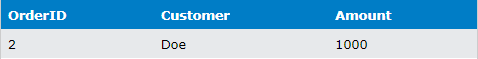To find customers whose names contain the substring 'oh':

``````SELECT *
FROM Orders
WHERE Customer LIKE '%oh%';

``````

Where statement in SQL output: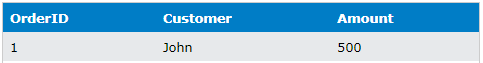## SQL WHERE AND

The SQL WHERE AND clause is used to combine multiple conditions in a SQL statement.

If we want to find orders made by John that are above \$500, we use the AND clause:

``````SELECT *
FROM Orders
WHERE Customer = 'John' AND Amount > 500;

``````

Since John's order amount is not more than \$500, the SQL clause where output would be empty.

## SQL WHERE OR

The SQL WHERE OR clause is used in SQL where you want to fetch records that satisfy at least one of the conditions.

To fetch orders made by John or orders above \$1000, we use the OR clause:

``````SELECT *
FROM Orders
WHERE Customer = 'John' OR Amount > 1000;

``````

The output of this SQL where clause would be: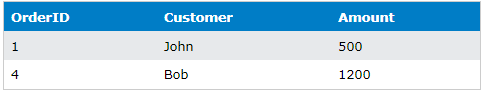## SELECT Query in SQL with Multiple WHERE Clause

We can also use multiple WHERE clauses in a SQL statement. The SELECT WHERE OR clause combined with the SQL WHERE AND clause allows us to filter records based on multiple conditions.

For example, if we want to select orders made by John or those made by others but above \$1000:

``````SELECT *
FROM Orders
WHERE Customer = 'John' OR (Customer != 'John' AND Amount > 1000);

``````

The output of this SQL where query:## SQL UPDATE with WHERE Clause

The SQL WHERE clause is also used with the UPDATE statement. The SQL UPDATE with WHERE clause allows us to update specific rows in a table.

Let's say we want to decrease the amount for John's order by \$100. The SQL query would be:

``````UPDATE Orders
SET Amount = Amount - 100
WHERE Customer = 'John';

``````

After executing the above SQL where query, John's order amount in the 'Orders' table would be \$400.## SQL UPDATE with SELECT in WHERE Clause

We can also use a SELECT SQL WHERE query in an UPDATE statement. This is known as a subquery. For example, if we need to increase the amount for the order with the lowest amount by \$200, the SQL query would be:

``````UPDATE Orders
SET Amount = Amount + 200
WHERE OrderID = (SELECT OrderID FROM Orders ORDER BY Amount ASC LIMIT 1);

``````

After executing the above SQL where query, the order with the lowest amount in the 'Orders' table would increase by \$200.

## SQL Case When

The SQL CASE WHEN statement is used to perform conditional logic and provide different output values based on specified conditions. It allows you to evaluate a series of conditions and return a result based on the first condition that is met.

Here's an SQL where query example using SQL CASE WHEN statement using the Orders table:

``````SELECT OrderID, Customer, Amount,
CASE
WHEN amount > 1000 THEN 'High'
WHEN amount > 500 THEN 'Medium'
ELSE 'Low'
END AS Amount_Category
FROM Orders;

``````

This query will return the Orderid, Customer, Amount, and Amount_Category for each row in the table.

### Final Words:

Where Clause in SQL is an essential part of SQL that allows us to filter and manipulate data effectively. From simple SQL WHERE query to complex situations involving the SQL WHERE IN Clause, understanding and using the WHERE clause can significantly enhance your data manipulation capabilities.